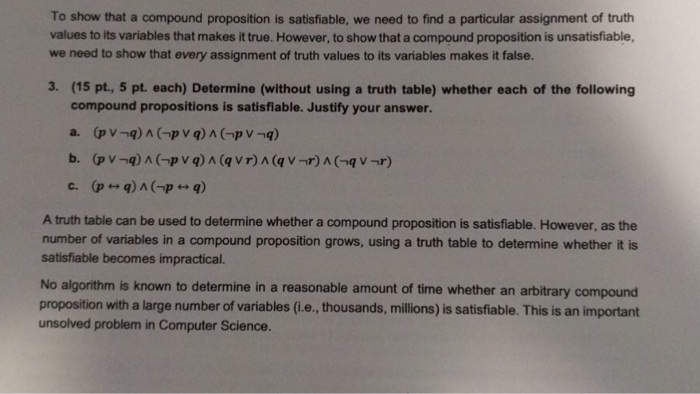# Answered Essay: To show that a compound proposition is satisfiable, we need to find a particular assignment of truth values to its variables that makes it true. HTo show that a compound proposition is satisfiable, we need to find a particular assignment of truth values to its variables that makes it true. However, to show that a compound proposition is unsatisfiable, we need to show that every assignment of truth values to its variables makes it false. Determine (without using a truth table) whether each of the following compound propositions is satisfiable. Justify your answer. a. (p q) Lambda (p q) Lambda (p q) b. (p q) Lambda (p q) Lambda (q r) Lambda (q r) Lambda (q r) c. (p doubleheadarrow q) Lambda (q doubleheadarrow q) A truth table can be used to determine whether a compound proposition is satisfiable. However, as the number of variables in a compound proposition grows, using a truth table to determine whether it is satisfiable becomes impractical. No algorithm is known to determine in a reasonable amount of time whether an arbitrary compound proposition with a large number of variables (i.e., thousands, millions) is satisfiable. This is an important unsolved problem in Computer Science.

3
a.
(p or NOT q) AND (NOT p or q) is true either when p = 1 and q = 1 or when p = 0 and q = 0 i.e p = q

now to (NOT p OR NOT q) is true when either p or q or both are 0

so if and only if p and q both are zero/false, the equation is true

b.
(q or r) AND (NOT q or NOT r) is true if and only if q is not equal to r

(q or NOT r) and also considering the previous condition, to get true value, q must be true and r must be false

so till now conclusion–> q = true, r = 0

as said in part a
(p or NOT q) AND (NOT p or q) is true either when p = 1 and q = 1 or when p = 0 and q = 0 i.e p = q

given q = true, p should also be true.

so the equation is satisfiable if p = q = true, and r = false

c
(p <-> q) is true is p and q are equal, but
(NOT p <-> q) is true is p and q are not equal

since they contradict each other, the statement cannot be true.Calculate your paper price
Pages (550 words)
Approximate price: -

Help Me Write My Essay - Reasons:Best Online Essay Writing Service

We strive to give our customers the best online essay writing experience. We Make sure essays are submitted on time and all the instructions are followed.Our Writers are Experienced and Professional

Our essay writing service is founded on professional writers who are on stand by to help you any time.Free Revision Fo all Essays

Sometimes you may require our writers to add on a point to make your essay as customised as possible, we will give you unlimited times to do this. And we will do it for free.Timely Essay(s)

We understand the frustrations that comes with late essays and our writers are extra careful to not violate this term. Our support team is always engauging our writers to help you have your essay ahead of time.Customised Essays &100% Confidential

Our Online writing Service has zero torelance for plagiarised papers. We have plagiarism checking tool that generate plagiarism reports just to make sure you are satisfied.Our agents are ready to help you around the clock. Please feel free to reach out and enquire about anything.

Try it now!

## Calculate the price of your order

Total price:
\$0.00

How it works?

Follow these simple steps to get your paper doneFill in the order form and provide all details of your assignment.Proceed with the payment

Choose the payment system that suits you most.Receive the final file

Once your paper is ready, we will email it to you.

HOW OUR ONLINE ESSAY WRITING SERVICE WORKS

Let us write that nagging essay.## Submit Your Essay/Homework Instructions

By clicking on the "PLACE ORDER" button, tell us your requires. Be precise for an accurate customised essay. You may also upload any reading materials where applicable.Pick A & Writer

Our ordering form will provide you with a list of writers and their feedbacks. At step 2, its time select a writer. Our online agents are on stand by to help you just in case.Editing (OUR PART)

At this stage, our editor will go through your essay and make sure your writer did meet all the instructions.